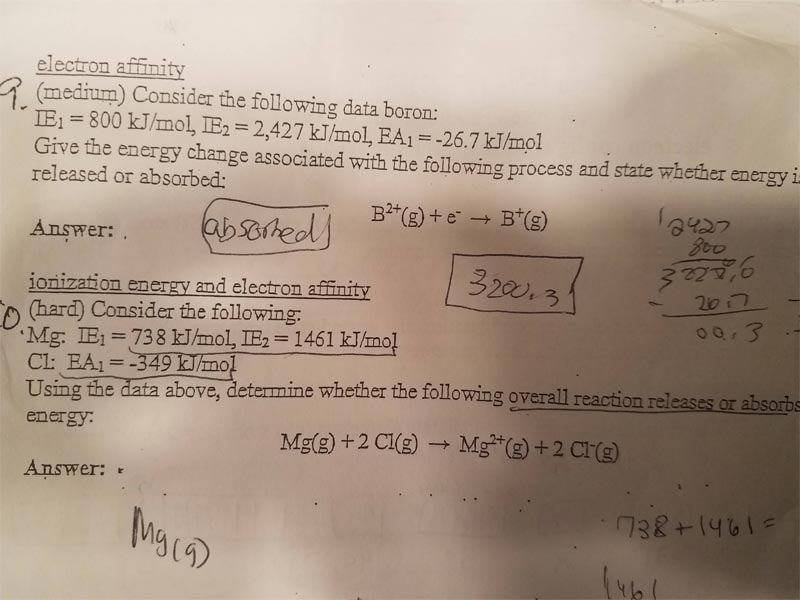# [College Chemistry] Electron Affinity and Ionization Energy

• Lori

## Homework Statement

Here's the pic of the problem and my work## The Attempt at a Solution

I'm not sure how I'm suppose to calculate the energy change? Did i do it correctly in the picture?

For number 9, I just added IE1 and IE2 and subtracted EA1 to get a total of 3200.3. This means that the atom would be absorbing energy right?

For number 10, like wise, i just added and subtracted for the IEs and EAs and got 1501 and it also means that it's absorbed

Honestly not quite sure what I'm doing . Can someone explain these 2 problems?

Hi Lori! :)

Ionization energy is the energy it takes to remove 1 (or more) electrons from a neutral atom, making it an ion.
Similarly, electron affinity is to add an electron to a neutral atom.

We start with B2+, for which we have spent 2427 kJ for a mol to make it ions.
That means it has 2427 kJ more (potential) energy than a neutral atom would have.
We end with 800 kJ for B+ on the right side.
So the change in energy is 2427-800=1627 kJ/mol.
Since we have more potential energy on the left side than on the right side, that energy has to go somewhere, which means it is released.

Last edited:
We start with Br2+, for which we have spent 2427 kJ for a mol to make it ions.
That means it has 2427 kJ more (potential) energy than a neutral atom would have.
We end with 800 kJ for Br+ on the right side.
So the change in energy is 2427-800=1627 kJ/mol.

I feel like it is wrong, looks to me like 2427 kJ is not energy required to remove two electrons, but only the second electron, so the 800 kJ number is completely irrelevant.

What the 9 probably wants you to do is to realize that adding an electron is a reverse reaction to the ionization, for example electron affinity of Fe3+ and ionization energy of Fe2+ are identical, they just have an opposite sign.

10 is not only about magnesium - for the reaction to take place you need magnesium to lose two electrons AND two chlorine atoms to capture electrons. Overall energy exchanged has to take it all into account.

•I like Serena
Nice catch!
Sorry Lori, the ionization energy is indeed the energy it takes to remove exactly 1 electron from a neutral atom or an ion.

Nice catch!
Sorry Lori, the ionization energy is indeed the energy it takes to remove exactly 1 electron from a neutral atom or an ion.
So, would it be 2427+800 kj spent on removing the two electrons? What do we use the EA for? I'm still confused on the whole concept :(!

What do we use the EA for?

Don't fall into a "if it is given it must be used" trap.

Don't fall into a "if it is given it must be used" trap.
Ahh. Okay. I under number 9. So basically the change in energy is just 2427 , and it is releasing energy because a electron is being add in the process.

However, I'm still confused on number 19. What is happening with chlorine? I know that magnesium is losing electrons so the energy is just 738 + 1461, but what is energy change for chlorine?

What happens to chlorine - does it lose an electron? Capture an electron?

The chlorine atoms go from neutral to negative, so that means that they are gaining electrons? Since EA is -349, it took 2 * -349 Kj to add a electron. Since it took 2199 Kj to remove electrons for the Mg, it takes -698 Kj to add the electrons. So the difference would then be 1501 kj change in energy? Also, since most of the energy is gained by removing electrons, would the process be absorbing energy?

The chlorine atoms go from neutral to negative, so that means that they are gaining electrons? Since EA is -349, it took 2 * -349 Kj to add a electron.

To add electrons. Two electrons to two atoms. But as you calculated it right below, perhaps that's just a typo.

And kJ, not Kj (watch the capitalization).

Since it took 2199 Kj to remove electrons for the Mg, it takes -698 Kj to add the electrons.

Not "since" Just "it took 2199 kJ to remove electrons for the Mg and it takes -698 kJ to add the electrons to Cl".

So the difference would then be 1501 kj change in energy? Also, since most of the energy is gained by removing electrons, would the process be absorbing energy?

Looks OK to me.

•Lori